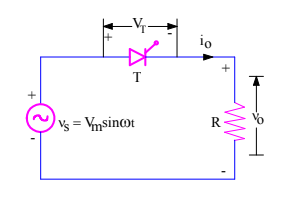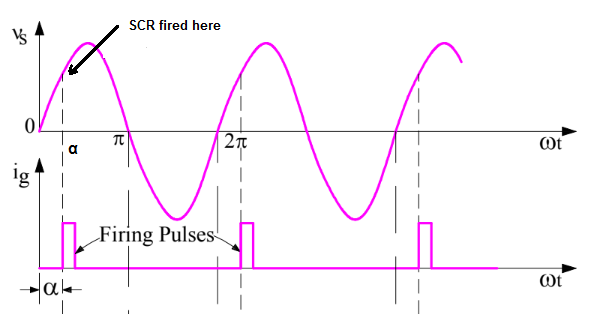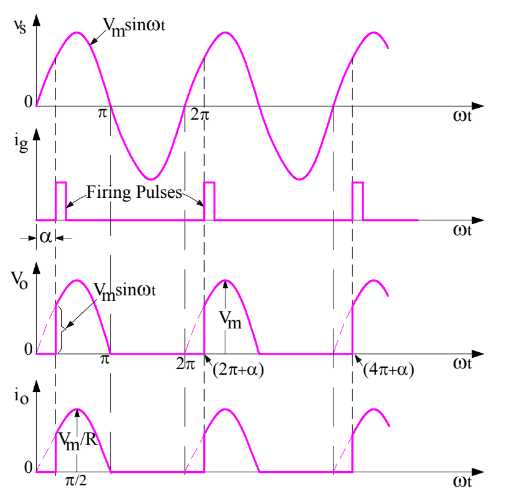# Phase Control of SCR – Definition, Explanation and Advantage

### Definition:

Phase Control of SCR means having control on the phase relationship between the start of current through the SCR and source voltage.

### Phase Control of SCR Explanation:

Whenever we talk of phase angle, we generally mean the angle of a sinusoidal quantity at any instant of time. Phase control of SCR means the phase angle (with reference to source voltage) where it is getting turned ON by the application of gate signal.

Let us understand the concept with the help of a simple circuit diagram as shown below.In the above circuit, a thyristor (or SCR) T is connected to load R and voltage source vs. This SCR will not conduct until and unless it is forward biased and gate signal is applied. Application of gate signal is called firing. You are requested to read “Firing Angle of SCR” to have clear understanding.

During positive half cycle of supply voltage vs, the SCR is forward biased. If the thyristor T is fired (say at some phase angle α on the source voltage), it will become ON.As SCR is now ON, it will start conducting. It will conduct from ωt = α to π. Since load is resistive in nature, the load voltage v0 and load current i0 will follow the waveform of supply voltage. The load voltage, load current and supply voltage waveforms are shown in figure below.Compare the waveform of source voltage vs and load current i0. You will observe that, the SCR is getting turned ON at a phase angle of α. Thus phase angle where thyristor T starts conducting is dependent on firing angle. If firing angle α is 0 degree, then load current and source voltage will be in phase whereas if α is 90 degree then load current will start when the source voltage is maximum. Thus the starting phase angle of load is controlled by firing angle. This must not be confused with the phase difference between the source voltage and load current.

At ωt= π, thyristor T will get commuated as the load current becomes zero and SCR is reversed biased from ωt = π to 2π. This is known as Natural Commutation. We again need to fire SCR at (2π+α), (4π+α), (6π+α) and so on.

### Advantage of Phase Control of SCR:

By having a phase control of SCR, we can have a control on output or load voltage. Just think, if the thyristor T is fired at α = 0 degree, the average load voltage would have been maximum as the SCR is ON for wt = 0 to π. Similarly, if the firing angle α = π, the average load voltage would have been zero as SCR will not conduct at all. Thus, by phase control of SCR, we can control the average load voltage.

This site uses Akismet to reduce spam. Learn how your comment data is processed.